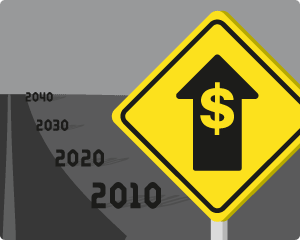# Planning for Retirement? Don’t Forget About InflationAnswering the question "How much do I need to save for retirement?" requires you to estimate how much you think you could live on after you retire. But, don't forget about inflation.

If you think you could live on \$75,000 based on today's prices, what would that be equivalent to 20 years from now? We can't predict exactly what inflation will be in the future, but that doesn't mean you shouldn't include inflation in your planning.

For example, let's say you plan to retire in 20 years. If we assume that inflation will be an annual 2%, then to maintain the same spending habits that a yearly income of \$75,000 affords you today, your starting income at retirement would need to be \$111,446.

So, I hope you can see why it's important to consider inflation when you're planning for retirement. If you are using the Retirement Annuity Calculator, this is something you'll need to consider.

You can use our simple Inflation Calculator to try some of your own calculations. For the example above, you'd enter 75000 as the Present Value, 20 years, and 2% for the rate, then press Calculate. The calculations are also pretty simple to do using Excel formulas. So, check out the Inflation Calculator page for information about the Excel formulas and to download a basic spreadsheet that demonstrates them.

## What Annual Inflation Rate Should I Assume?

Take a look at the graph below from wikimedia.org that shows the U.S. Inflation Rate.

To pick an average inflation rate to use for future estimates would require you to make some estimate about where you think the economy is headed. We're currently in a period of low inflation (1-2%), but inflation has frequently been over 5%. You could eyeball the above graph to pick a rough average inflation rate, but there is also a way to calculate an average.

First, let's use the Inflation Calculator from bls.gov to find out that \$50,000 in 1979 has the same buying power as \$147,752.75 does in 2009. That calculation uses historical inflation rates.

To calculate an average annual inflation rate for that period using the Vertex42 Inflation Calculator, enter \$50,000 as the Present Value, \$147,752.75 as the Future value, and 30 for the number of years. The answer is 3.677%.

This approach is a lot easier than calculating the geometric mean directly from the historical data. Below are a few more historical average inflation rates.

From To Years Rate
1969 2009 40 4.51%
1979 2009 30 3.68%
1989 2009 20 2.78%
1999 2009 10 2.56%

So, based on these calculations, my use of 2% in the example above might actually be a little too optimistic.